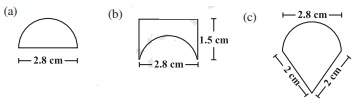# An ant is moving around a few food pieces of different shapes scattered on the floor. For which food-piece would the ant have to take a longer round? Remember, circumference of a circle can be obtained by using the expression C = 2πr ,where r is the radius of the circle(a)

Radius = Diameter/2 = 2.8/2 cm = 1.4 cm

Circumference of semi-circle = ∏.r = (22/7)×1.4 = 4.4

Circumference of semi-circle is 4.4 cm

Total distance covered by the ant = Circumference of semi – circle + Diameter

= 4.4 + 2.8

= 7.2 cm

Total distance covered by the ant = 7.2cm

(b)

Diameter of semi-circle = 2.8 cm

Radius = Diameter/2 = 2.8/2 = 1.4 cm

Circumference of semi-circle = r = (22/7) × 1.4 = 4.4 cm

Total distance covered by the ant= 1.5 + 2.8 + 1.5 + 4.4 = 10.2 cm

(c)

Diameter of semi-circle = 2.8 cm

Radius = Diameter/2 = 2.8/2 = 1.4 cm

Circumference of semi-circle = pi.r = (22/7) × 1.4 = 4.4 cm

Total distance covered by the ant = 2 + 2 + 4.4 = 8.4 cm

After analyzing results of three figures, we came to a conclusion that for figure (b) food piece, the ant would take a longer round.